﻿ Iron Loss of Coil (AC Analysis with B-H Curve Taken into Account)Examples | Product | Murata Software Co., Ltd.Example8Iron Loss of Coil (AC Analysis with B-H Curve Taken into Account)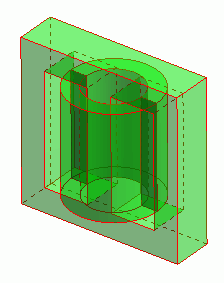General

• A coil is wound on a core. The iron loss is analyzed.

• Permeability is set with B-H curve table.
The effect of magnetic saturation is taken into account in this exercise whereas it is not taken into account in the [Exercise 29: Heating due to the Iron Loss] of magnetic field harmonic analysis.

• The core loss, the vectors of the magnetic field and the magnetic flux density are solved.

• Unless specified in the list below, the default conditions will be applied.

• See Exercise 2 regarding the calculation of heating due to the iron loss.

Analysis Space

 Item Setting Analysis Space 3D Model unit mm

Analysis Conditions

 Item Setting Solver Magnetic Field Analysis [Luvens] Analysis Type Transient Analysis Options None

The Transient Analysis tab is set up as follows.

50KHz AC is input. To reproduce its waveform well, the time step is set by dividing the periodic time of 50KHz by 12.

Tab

Setting Item

Setting

Transient Analysis

Time step

Automatic

Table

 Number Calculation steps Output steps Time step [s] 1 12 1 1.0/(12*50e3)

Graphical Objects

A coil is wound on a core.

The model is defined by the core (Core) and the loop coil (Coil) are defined.

The loss characteristics are defined in the iron loss table.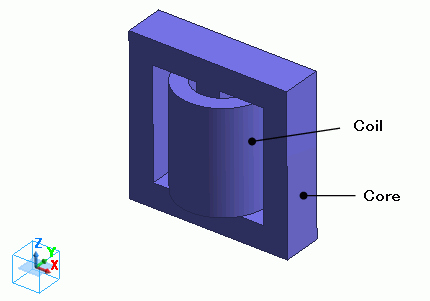Body Attributes and Materials

 Body Number/Type Body Attribute Name Material Name 5/Solid Coil 008_Cu * 6/Solid Core Core

(*) Available from the Material DB

The material properties of the core are set up as follows.

The permeability is defined with B-H curve table.

The loss characteristics are defined with the iron loss table.

Material Name

Tab

Setting

Core

Permeability

Magnetization Characteristic Type: Select B-H curve

B-H Curve Table

 Magnetic Field [A/m] Magnetic Flux Density [T] 0 0 58 0.219 90 0.33 180 0.59 380 0.69 1100 0.75 2000 0.78 3000 0.8 11000 0.9 20000 0.95

Electric Conductivity

Conductivity Type: Conductor

Conductivity: 0.1[S/m]

Iron Loss

Iron Loss Calculation Type: Iron loss table

Frequency: 5×10^4[Hz]

[Magnetic flux density-Loss density] Table *

 Magnetic Flux Density [T] Joule Loss Density [W/m^3] 50×10^-3 5×10^3 500×10^-3 500×10^3

* This is not the actual material’s property.

Press the Graph button. The following graph will show up.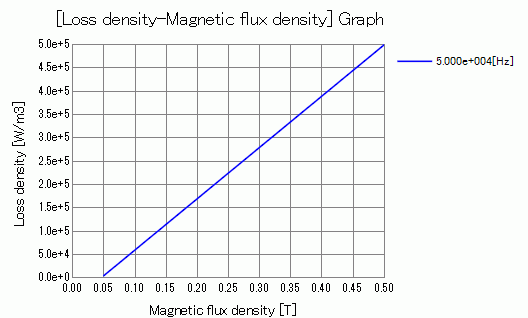Body attribute is set up as follows to apply current to the loop coil.

 Body Attribute Name Tab Setting Coil Current Waveform: AC Current: 0.1[A] Frequency: 50×10^3[Hz] Turns: 100[Turns] Direction: Loop Coil/Magnetic Field Direction Direction Vector of Magnetic Field: X=0, Y=0, Z=1

No setting.

Results

To see the iron loss, go to the [Results] taband click [Table]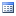.

The joule loss is shown below.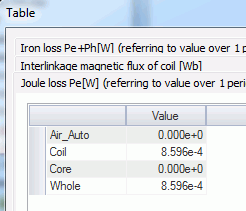The iron loss is shown below.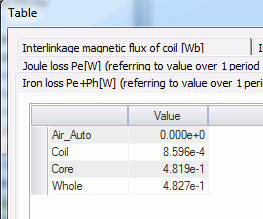The core’s iron loss is approximately 0.5W.

The joule loss and the hysteresis loss of the core are not given because the the material property is defined on the iron loss table.

The loss characteristics are defined in the iron loss table. Only iron loss is obtained.

If it is defined by the iron loss experimental formula, the joule loss and the hysteresis loss will be output as well.

See [Loss Calculation in the Magnetic Field Analysis] for details of the loss.

The vectors of the magnetic field are shown below.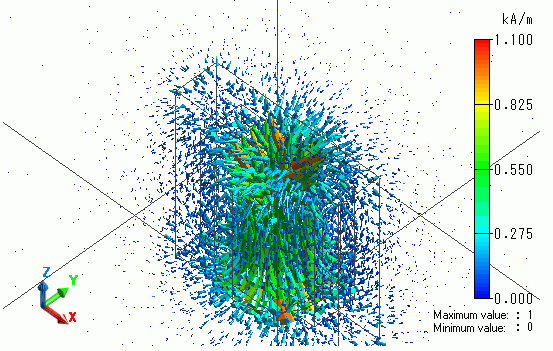The vectors of the magnetic flux density are shown below.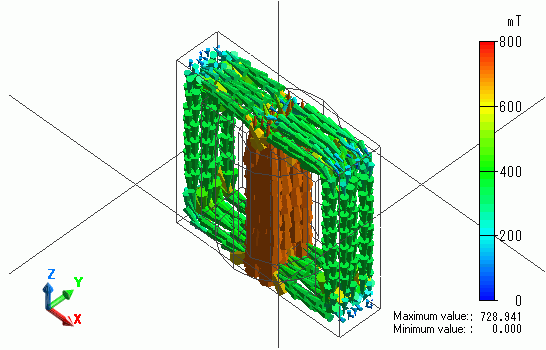The flux density is looping through the core.

The iron loss density is shown below.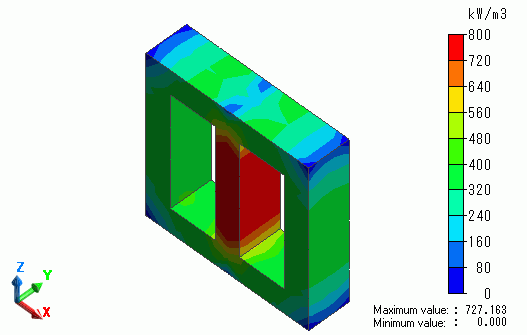﻿